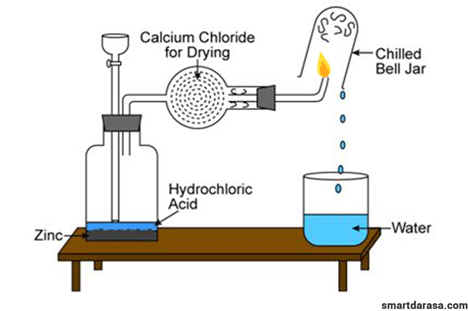• Data:

Mass of hydrogen gas (H2) = 2g
Molar volume at room temperature and pressure = 24 dm3
Asked: volume of water• Chemical equation for the reaction.

2H2(g) + O2(g) 2H2O(L)
Mole ratio 2:1:2
• To convert 2g of H2 into moles then to relate

Mole = mass/Molar mass
= 2g / 2g/mole
= 1 mole of H2

Mole equivalent between H2 and water.
2moles of H2 Ξ 2 moles of H2O
1mole of H Ξ ?? of H2
= (1 moles × 2 moles) / 2 moles
= 1 mole of H2

• To convert 1 mole of H2O into volume of water.

Mole = volume / Molar volume
Volume = mole × molar volume
= 1 mole × 24dm3/mole
= 24dm3

24dm3 of water was produced at S.T.P.